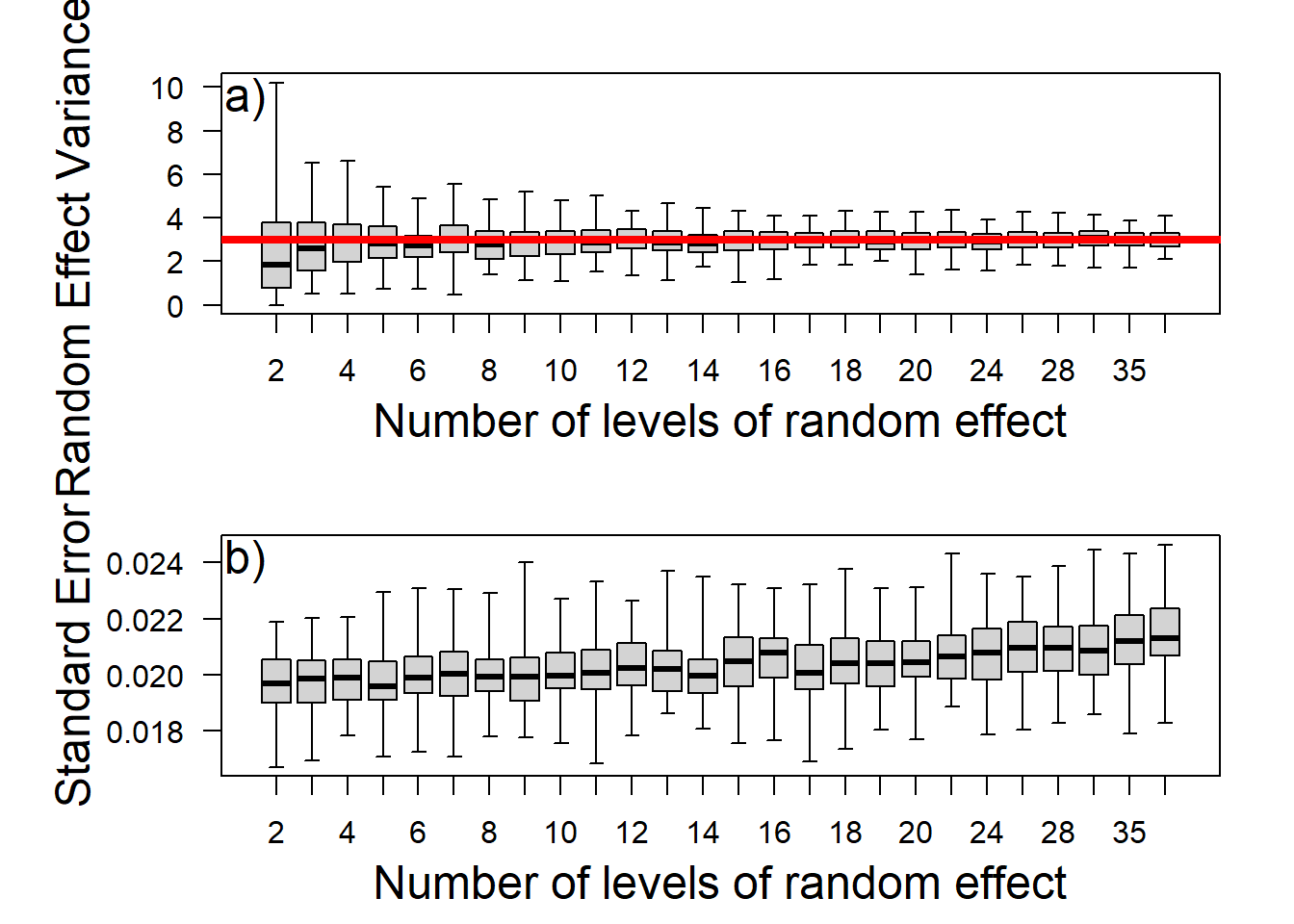Peril 3: Number of levels of the random effect

library(lme4)

Define parameters used in the simulations

#Number of grouping levels
groups<-c(seq(2,20,1),22,24,26,28,30,35,40)
#Total sample size
n<-200
#Standard deviation of the random effects distribution
gr.dev<-3
#Population intercept
intercept<-50
#Standard deviation for the residual deviance
resid.dev<-3
#Effect of the covariate
x.eff<-1

#Values of the explanatory variable
x<-round(runif(n,min=-20,max=20))

#Number of simulations to be conducted
reps<-100

Now we create empty matrices to store the results

rvar<-matrix(NA,nr=reps,nc=length(groups))
int_est<-matrix(NA,nr=reps,nc=length(groups))
x_est<-matrix(NA,nr=reps,nc=length(groups))
int_err<-matrix(NA,nr=reps,nc=length(groups))
x_err<-matrix(NA,nr=reps,nc=length(groups))

Now we can run the simulations

NB. We are suppressing the warning messages from model fitting from being printed here for presentation purposes. We would recommend re-running after deleting warning=FALSE and message=FALSE from the line below this to see what warnings arise and when they do if you are interested.

#loop over number of simulations
for(r in 1:reps){

#loop over the number of random effect levels
for(i in 1:length(groups)){

#simulate group data
group<-sample(1:groups[i],200,replace=TRUE)
group.means<-rnorm(groups[i],mean=0,sd=gr.dev)

#Simulate response variable
y<-numeric()
for(j in 1:n){
y[j]<-rnorm(1,intercept+x.eff*x[j]+group.means[group[j]],resid.dev)
}

#Fit mixed model in lme4
mod<-lmer(y~x+(1|group))

#Quantify model estimates that we want to keep
rvar[r,i]<-sqrt(unlist(VarCorr(mod)))
int_est[r,i]<-fixef(mod)
x_est[r,i]<-fixef(mod)
int_err[r,i]<-summary(mod)$coefficients[1,2] x_err[r,i]<-summary(mod)$coefficients[2,2]

} #end groups loop
} #end reps loop

Now we can plot the results, as used in the main text

par(mfrow=c(2,1),mar=c(4,6,2,2))
boxplot(rvar,col="light grey",range=0,las=1,lty=1,names=groups)
lines(x=c(-20,100),y=c(3,3),lwd=4,col="red")
mtext("Random Effect Variance (SD)", side=2,line=3.4,cex=1.5)